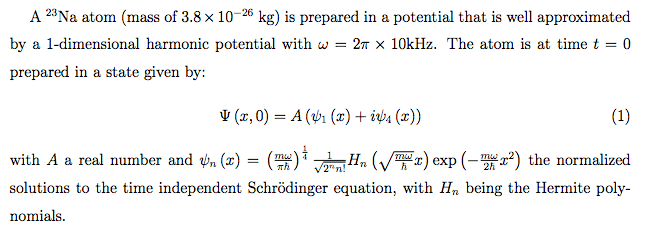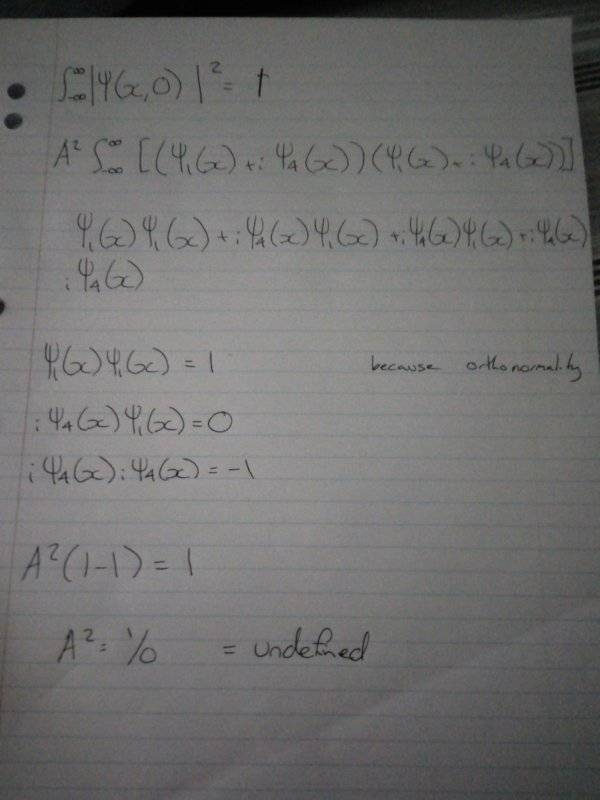# Normalization constant A of a harmonic oscillator

Sorin2225
Homework Statement:
Finding the normalization constant A of a harmonic oscillator
Relevant Equations:
(psi(x,t))^2=1I've worked through it doing what I thought I should have done. I normalized the original wavefunction(x,0) and made it = one before using orthonormality to get to A^2(1-1) because i^2=-1 but my final answer comes out at 1/0 which is undefined and I don't see how that could be correct since A is meant to be a real number. I'm not really sure where I've gone wrong either so any insight would be appreciated.

Staff Emeritus
Homework Helper
##\lvert \psi_1 + i \psi_4 \rvert^2 \ne (\psi_1 + i \psi_4)^2##

The vertical lines matter.

Sorin2225
So it's the absolute value? which means that it's +1 not -1?

Staff Emeritus
Homework Helper
What is “it”?

Sorin2225
|iψ4*iψ4|

Staff Emeritus
Homework Helper
That's not how absolute values work. You can't say ##\lvert a+b \rvert = \lvert a \rvert + \lvert b \rvert## in general.

How do you calculate the modulus of a complex number?

Sorin2225
sqrt(a^2+b^2) so it would be Sqrt((i^2)^2+((psi(4)^2)^2))